# Pyramids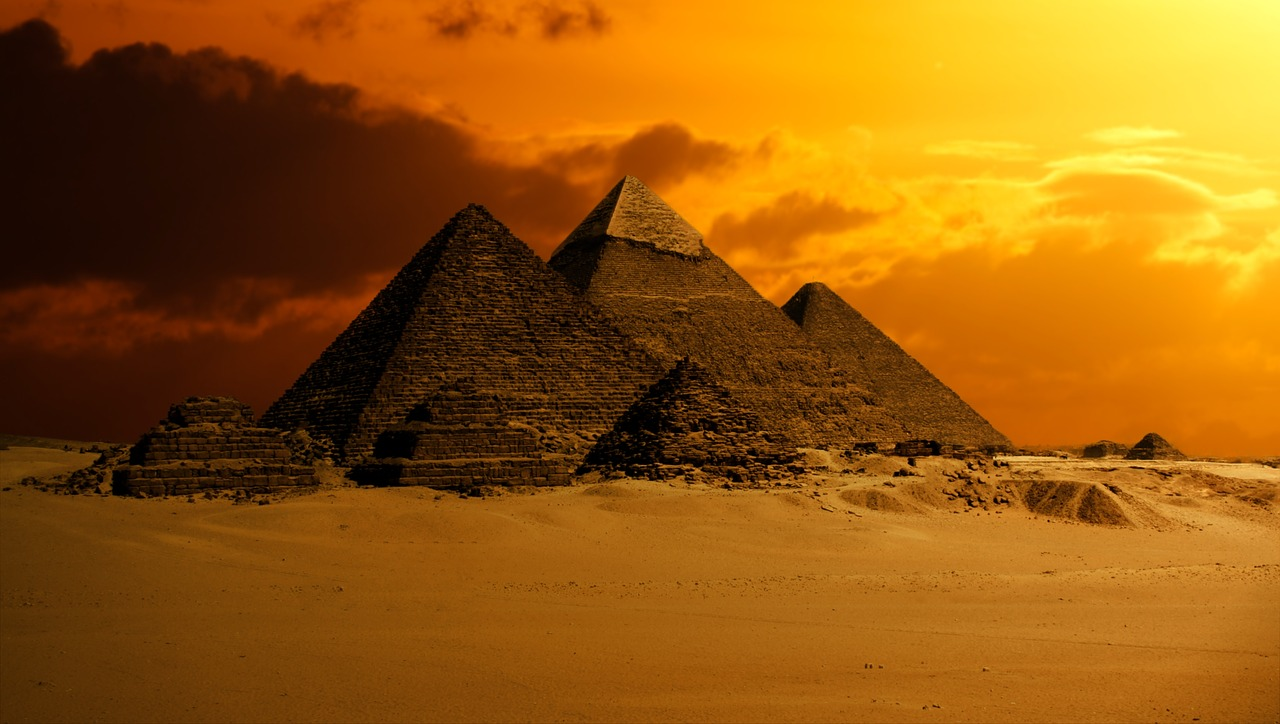When we think about pyramids, the pyramids of Egypt or the Mayan pyramids of Mexico spring to mind. These structures have fascinated people for centuries. But a pyramid is a general term for a solid that has a polygon as a base, and sides that slope up to meet at a single point. The Egyptian and Mayan pyramids are modelled on square pyramids: pyramids that have a square as their base.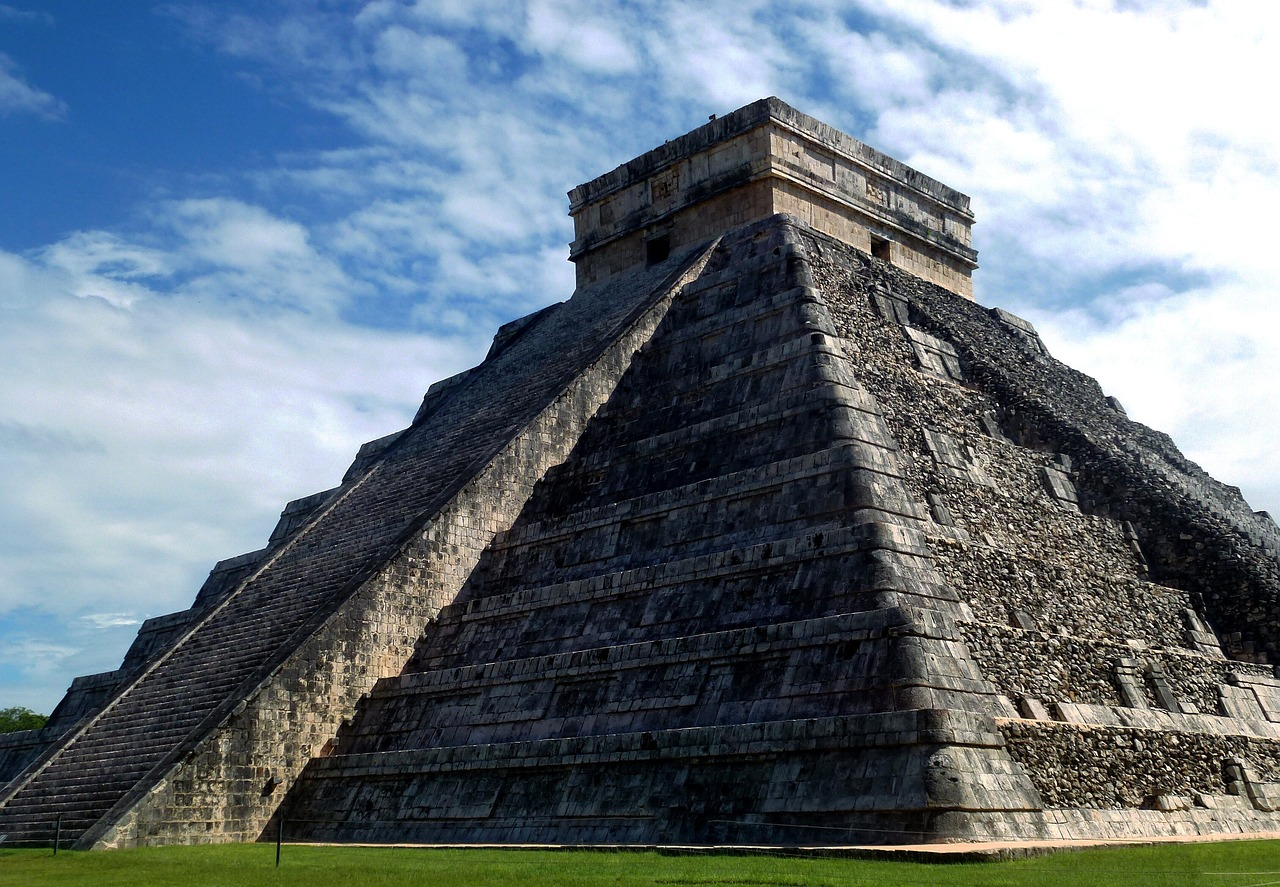## The Structure of a Pyramid

Pyramids are solids formed by connecting a polygon, called the base to a single point, called the apex as shown in the picture below.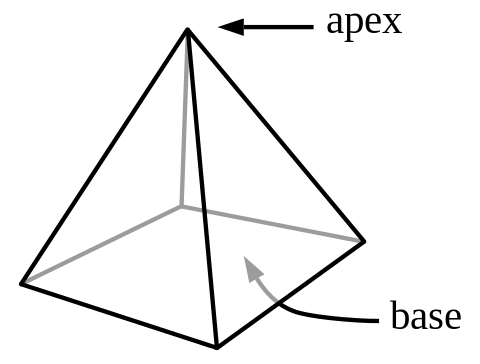The most common pyramids have triangles or squares as their bases, but pyramids can have any polygon as their base.

## Different Kinds of Pyramids

There are many different kinds of pyramids. They are named after the polygon that forms their base. Here are a few different examples.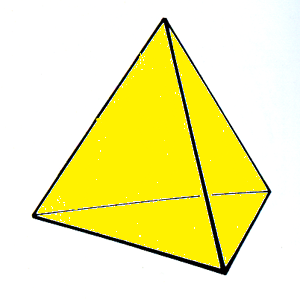A triangular pyramid is also known as a tetrahedron. It has four faces, all shaped like triangles. It also has four vertices.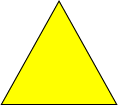This is a square pyramid. Its base is shaped like a square, and it has four sloping faces, all shaped like triangles. It has five vertices.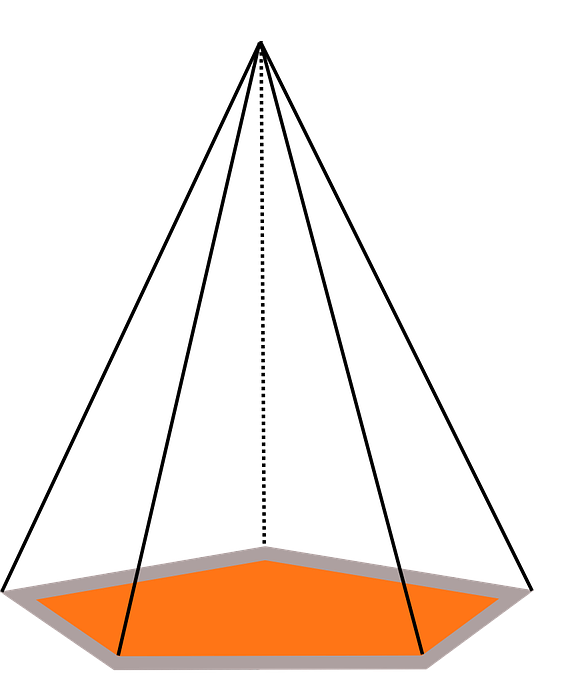This is a pentagonal pyramid. Its base is shaped like a pentagon, and it has five sloping faces, all shaped like triangles. It has six vertices.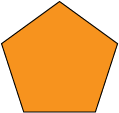This is a hexagonal pyramid. Its base is shaped like a hexagon, and it has six sloping faces, all shaped like triangles. It has seven vertices.Of course, we can build pyramids with heptagons, octagons, nonagons, decagons etc as bases. The possibilities are endless.

## Some Other Words Used to Describe Pyramids

### Right and Oblique Pyramids

Right pyramids are pyramids in which the apex is directly above the centre of the base like the one in this picture.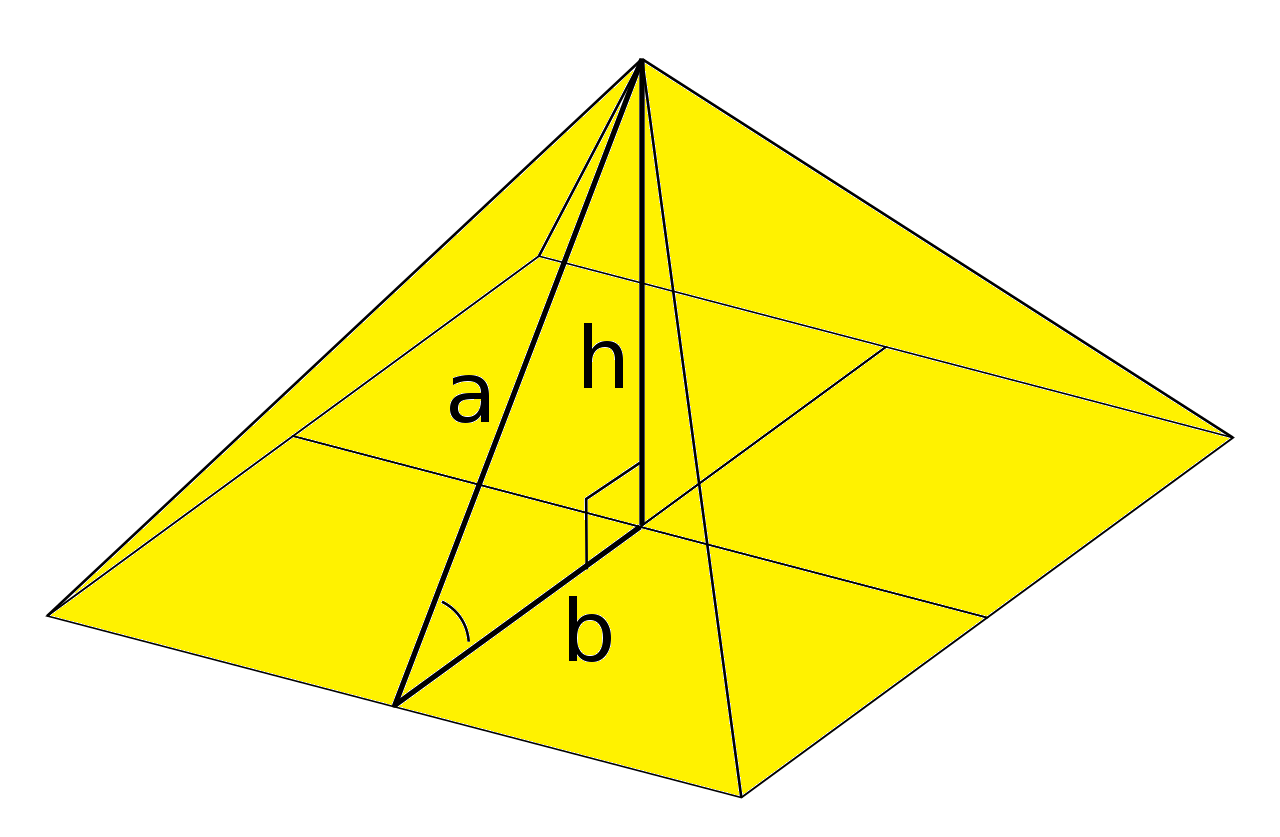Oblique pyramids are pyramids in which the apex is not directly above the centre of the base like the one in this picture.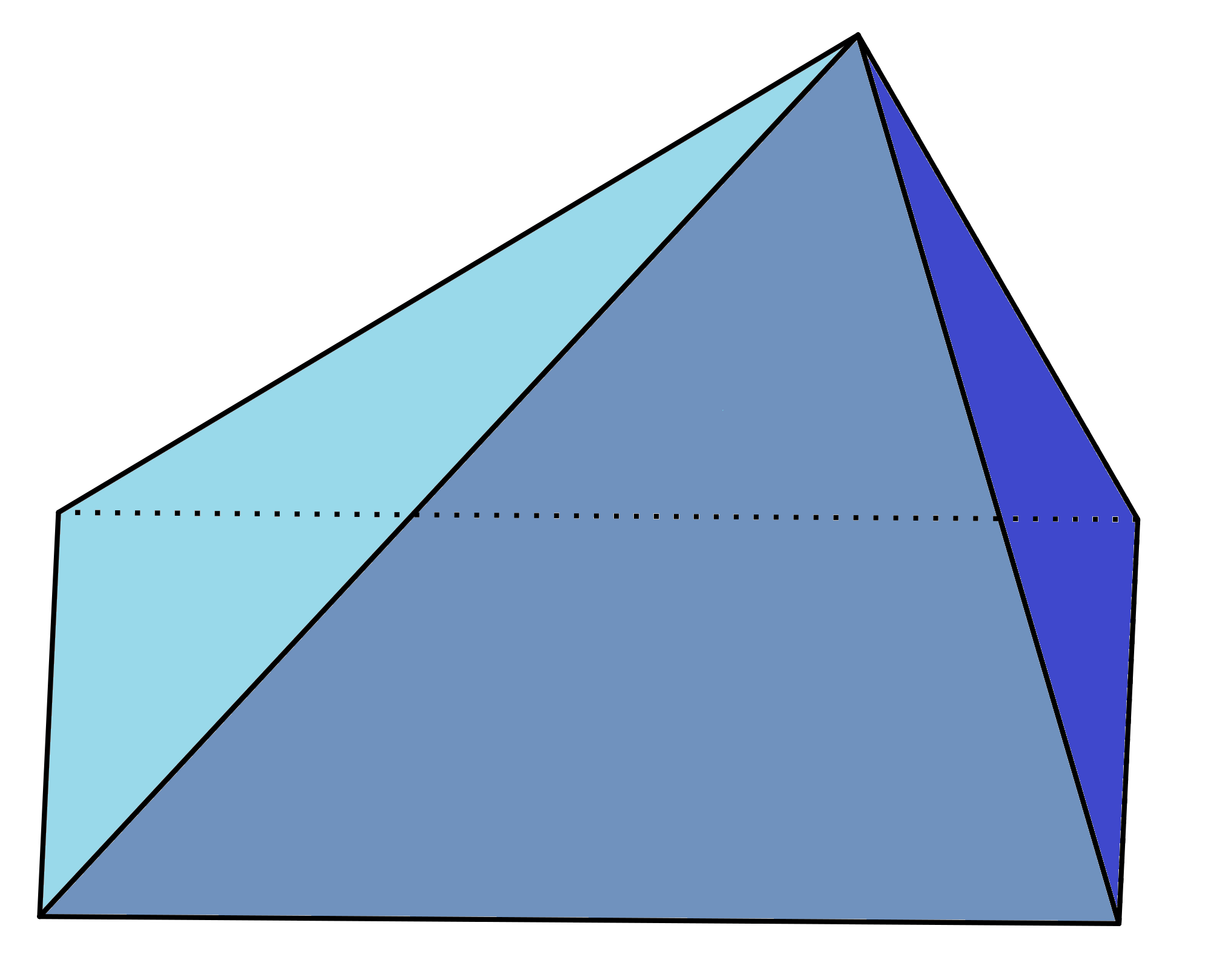The Ancient Egyptian pyramids were essentially right square pyramids (some of them were a little off).

### Regular and Irregular Pyramids

Regular pyramids have regular polygons (ones with all equal sides and angles) as their bases. Irregular pyramids have irregular polygons as their bases.

All of the pyramids shown in the section on different types of pyramids are regular pyramids. The pyramid in the picture below is irregular. Its base is an irregular hexagon.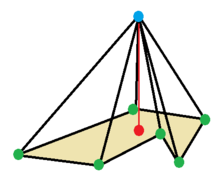## Surface Area and Volume of Pyramids

The volume of a pyramid is one third of the volume of a prism having the same base and height:

$V = \dfrac{1}{3} \times (\text{Area of Base}) \times \text{Height}$

The surface area of a pyramid can be calculated in two parts:

1. The base area (the area of its base)
2. The lateral area (the sum of the areas of the sloping sides)

These are added together to give the surface area of the pyramid. So, the surface area of a pyramid is given by the formula

$SA =(\text{Area of Base}) + ( \text{Lateral Area})$

If all of the side faces are the same, we can simplify the formula to give:

$SA =(\text{Area of Base}) + \dfrac{1}{2} \times (\text{Perimeter of Base}) \times ( \text{Slant Height})$

The slant height is the height of each sloping triangular side, which is labelled by $s$ in the picture below:### Description

There are several lessons related to 3D geometry such as

1. Euler's formula
2. Vertices, Edges and Faces
3. Volumes of 3D shapes
4. etc

Even though we've titled this lesson series to be more inclined for Year 10 or higher students, however, these lessons can be read and utilized by lower grades students.

### Prerequisites

Understanding of 3D shapes

### Audience

Year 10 or higher, but suitable for Year 8+ students

### Learning Objectives

Get to know 3D Geometry

Author: Subject Coach
You must be logged in as Student to ask a Question.